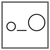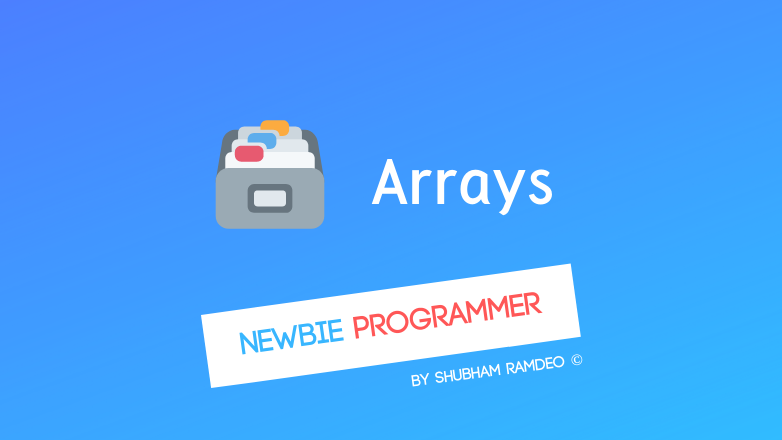Fuddlepixel coz tech is fun();

# Introduction to Arrays

A brief introduction of arrays in C. How arrays are created and used to store data as a table. What is the difference between arrays and variable ?

By Shubham Ramdeo
28 February 2016 · 5 mins read
# `c`  `newbie`That’s how data table can be made, use arrays !

Welcome to the Newbie Programmer series. With the last post, we have ended a big discussion on the program structure. Now let us start a new topic. So if you are new to this series, please go to the index (click here) and read out all the previous parts so that you can easily understand what is going on down below.

Let’s start with Arrays. Arrays are also a kind of variables but are created and used in a different manner.

We have supposed variable as a bag, or a box. A box containing different kinds of balls. That is a simple variable as we have used so far. But now suppose a Big box, containing many small partition. And in each of those partition, you can store balls. That is an array. This example of box and ball is illustrated in this image below.So Array means table. For example, let’s make a student roll number table, holding the data of their attendance percentage.

``````Student's Roll Number Attendance
1                     78
2                     64
3                     87
4                     92
5                     76
``````

So here we have 5 students, and each student is having their attendance value. How can we use such tables during programming in an easy way? Use arrays.

So here we will write :

``````int attend = { 78, 64, 87, 92, 76 };
``````

The above line will create a table, or now we can say an array. The name of this array is ‘attend’. It is holding the integer values. As we have 5 students so the size of this array is 5. The data are listed in order within { and }.

The thing is that in C language, arrays starts from zero.

So here when we use :

``````printf("%d", attend);
``````

We will get 78.

And if

``````printf("%d", attend);
``````

We will get 64.

Here “the point to be noted” is that the size of the array is 5, but attend do not exist. These 5 values are : attend, attend, attend, attend and attend.

Let’s extend this example. Suppose we want to make a program that uses an array to store attendance percentage of 10 students. And in the school, students below 75% attendance will not give exams. So let us find out which roll numbers are not allowed.

We can use loop for that.

``````#include <stdio.h>
main()
{
int attend ={78, 64, 87, 92, 76, 63, 89, 83, 91, 77};
int count;
for(count = 0; count <10; count++)
{
if(attend[count] < 75)
{
printf("Roll number %d is not allowed.\n", count+1);
}
else
{
printf("Roll number %d is allowed.\n", count+1);
}
}
}
``````

OUTPUT :

``````Roll number 1 is allowed
Roll number 2 is not allowed
Roll number 3 is allowed
Roll number 4 is allowed
Roll number 5 is allowed
Roll number 6 is not allowed
Roll number 7 is allowed
Roll number 8 is allowed
Roll number 9 is allowed
Roll number 10 is allowed
``````

Working :

1. An array is created, ‘attend’, with size 10, and each of it’s columns are stored with the data given.
2. A variable ‘count’ is created for the purpose of ‘for loop’. So it will check the data stored in attend[count]. If this value is less than 75, the message of “not allowed” will be printed. Otherwise message of “allowed” will get printed.
3. Loop starts with count=0. So it checks attend which is more than 75 so the message of “is allowed” get printed and first loop ends.
4. Then count = 1. Again it get checked. This time attend is 64 which is less than 75 so the message of “not allowed” get printed.
5. This process continues till count = 9. Then program ends.
6. Look, here I have used count+1 in printf so as to remove the problem of arrays being starting from zero. Otherwise, the output will be printing “Roll number 0 is allowed…”

Here we have made an array like this :

``````data_type array_name[size] = { data };
``````

We can do without adding any data like :

``````data_type array_name[size];
``````

For example :

``````#include <stdio.h>
main()
{
int num;
num = 23;
num = 55;

printf("Enter a number for num\n");
scanf("%d",&num);
printf("The numbers inside num array : %d, %d, %d \n", num, num, num);
}
``````

In the above program we have made a an array of size 3, so we can have num, num and num to store values. We have already assigned the value of num and num. And then used scanf to ask the user to input its value.

In all the above examples, we have considered a single dimension array. We can create two and three-dimensional, even multidimensional arrays.

The above image you see is an example of 1 dimensional array. A TIC TAC TOE like table is an example of 2 dimensional array. Rubik’s cube is a typical example of 3 dimensional array.

So to make such multidimensional arrays, you can do something like :

``````data_type array_name[size of first dimension][size of second dimension]...[size of nth dimension];
``````

We can represent a tic tac toe position like :

``````x | O | O
O | X | O
X | O | X
``````

As array as :

``````char ttt = { 'X', 'O', 'O'
'O', 'X', 'O'
'X', 'O', 'X' };
``````

In this, we have created an array of 3 rows and 3 columns, and then given the data.

Here ttt is ‘X’.

So I think that’s enough for today. We have discussed a basic introduction of Arrays. In the next part, we will discuss some tricks and tips and some great examples of using arrays for practical life problems. If you have any doubt, just comment below or meet me at social sites.

So Stay connected :)

##### Update me weekly ✉

Subscribe below and we’ll send you a weekly email summary of all new Code tutorials. Never miss out on learning about the next big thing.

#### Shubham RamdeoShubham Ramdeo is the founder of Fuddlepixel. He is a geek, a writer, involved with many technical and humanity projects.

Meet me!

#### Recent#### Related

`c`

`newbie`

Top